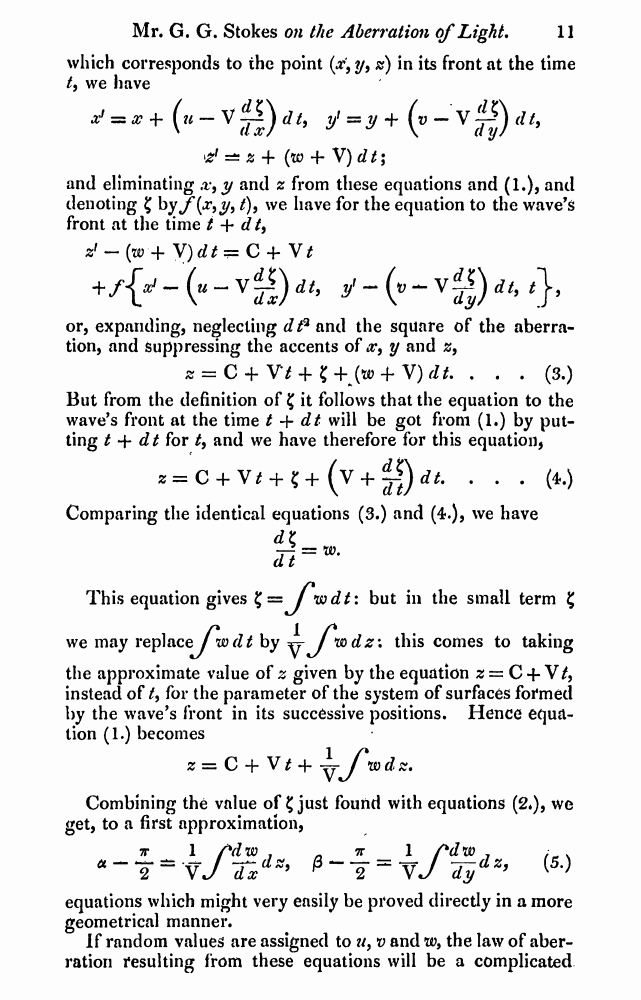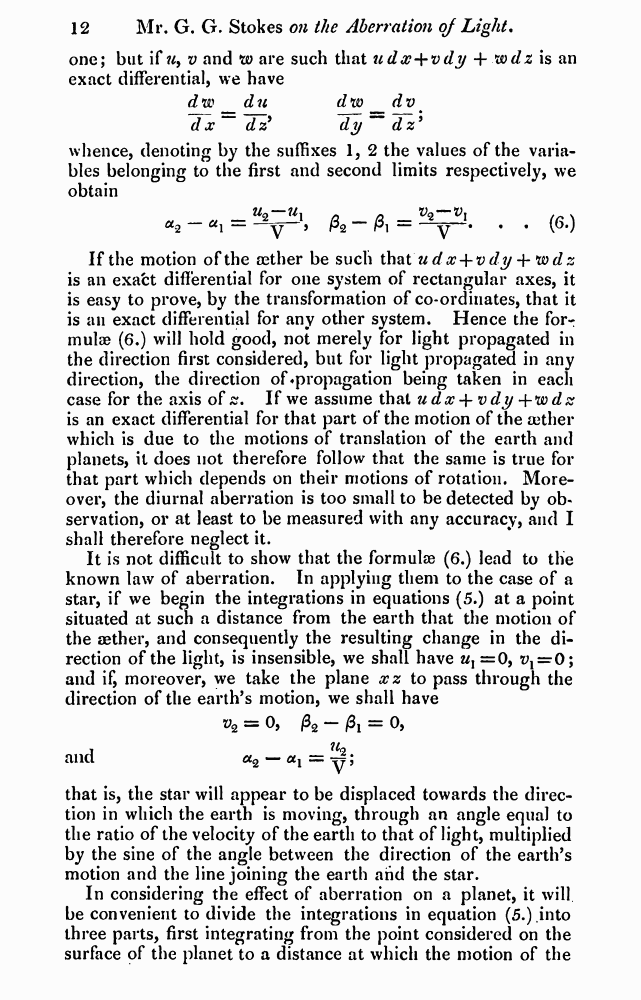﻿ G.G.Stokes "On the Aberration of Light" // Philosophical Magazine 1845, 27: Page 11

# G.G.Stokes "On the Aberration of Light" // Philosophical Magazine 1845, 27: Page 11

В начало   PDF, DjVu   <<<     Страница 11   >>>1  2  9  10  11 12  13  14  15

which corresponds to the point (x’9 y, z) in its front at the time t9 we have

*? = x+(u-V&)dt, y=jr+(B-v^)rf/,

d =± z + (to + V) d t;

and eliminating ,v9 y and z from these equations and (1.), and denoting £ hyf(x9 y9t)9 we have for the equation to the wave’s front at the time t + dt9

zf — {w + V)dt=C + V/

## +/{/ - (» - vg) it, y - (»- vg) i/, <},

or, expanding, neglecting dt3 and the square of the aberration, and Suppressing the accents of y and z9

z = C + Vt + 1'+^w + V)dt. . . . (3.)

But from the definition of £ it follows that the equation to the wave’s front at the time t + dt will be got from (1.) by putting t 4- dt for t9 and we have therefore for this equation,

z=C + V* + ?+ (V + ifydt. . . . (4.) Comparing the identical equations (3.) and (4.), we have

### <*K

77 = w-d t

This equation gives £= J”wdt: but in the small term £

we may replaceylwrZrf by ^ J*mdz\ this comes to taking

the approximate value of z given by the equation z = C + V/, instead of t9 for the parameter of the system of surfaces formed by the wave’s front in its successive positions. Hence equation (1.) becomes

z=C + V^-f—J*wdz.

Combining the value of £ just found with equations (2*), we get, to a first approximation,

7r 1 rdtxi J 7T 1 /*dw J : s

## * 2 ~ vJ dx * P 2 “ VJ dy ’ ^

equations which might very easily be proved directly in a more geometrical manner.

If random values are assigned to a9 v and w9 the law of aberration resulting from these equations will be a complicated

one; but if u> v and w are such that udx+vdy + wdz is an exact differentia], we have

dw d u dw^dv

d x~ dz9 dy~~dz9

whence, denoting by the suffixes 1, 2 the values of the variables belonging to the first and second limits respectively, we obtain

= . . (6.)

If the motion of the aether be such that udx + vdy -\-*wdz is an exafct differential for one system of rectangular axes, it is easy to prove, by the transformation of co-ordinates, that it is an exact differential for any other system. Hence the formulae (6.) will hold good, not merely for light propagated in the direction first considered, but for light propagated in any direction, the direction of ♦propagation being taken in each case for the axis of ss. If we assume that u dx + v dy + w dz is an exact differential for that part of the motion of the aether which is due to the motions of translation of the earth and planets, it does not therefore follow that the same is true for that part which depends on their motions of rotation. Moreover, the diurnal aberration is too small to be detected by observation, or at least to be measured with any accuracy, and I shall therefore neglect it.

It is not difficult to show that the formulae (6.) lead to the known law of aberration. In applying them to the case of a star, if we begin the integrations in equations (5.) at a point situated at such a distance from the earth that the motion of the aether, and consequently the resulting change in the direction of the light, is insensible, we shall have ux =0, ^=0; and if, moreover, we take the plane xz to pass through the direction of the earth’s motion, we shall have = 0, /32 = 0,

and ct2 — &i = —;

that is, the star will appear to be displaced towards the direction in which the earth is moving, through an angle equal to the ratio of the velocity of the earth to that of light, multiplied by the sine of the angle between the direction of the earth’s motion and the line joining the earth and the star.

In considering the effect of aberration on a planet, it will be convenient to divide the integrations in equation (5.) into three parts, first integrating from the point considered on the surface of the planet to a distance at which the motion of the